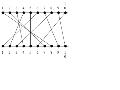# sopherwenxin学习笔记

posts - 5, comments - 8, trackbacks - 0, articles - 11

10

8  7  4  2  5  1  9  3  10  6

1

22

### Feedback

To hnhls99 :

To 学习笔记：

#include<stdio.h>
#include<malloc.h>
void main()
{
int n,m,i,j,k,count=0;
int *a;
scanf("%d",&m);
for(i=0;i<m;i++)
{
scanf("%d",&n);
a=(int *)malloc(n*sizeof(int));
for(i=0;i<n;i++)
{
scanf("%d",&a[i]);
}
for(j=0;j<n;j++)
{
for(k=j+1;k<n;k++)
{
if(a[j]<a[k]) count++;
}
}
printf("%d\n%d\n",i,count);
}

}

 只有注册用户登录后才能发表评论。 【推荐】超50万行VC++源码: 大型组态工控、电力仿真CAD与GIS源码库 相关文章: